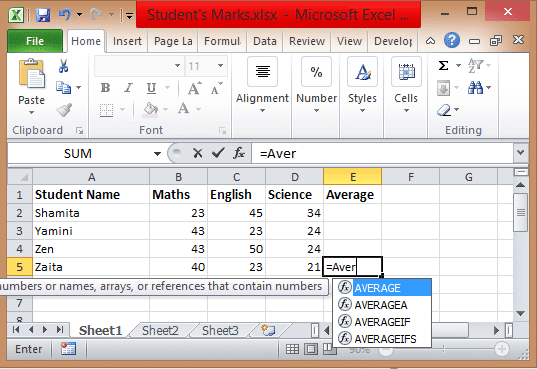To advertise with us contact on Whatsapp: +923041280395 For guest post email at: [email protected]

# Excel Formulas Basic Functions like Sum, AverageExcel Formulas Basic Function: The formula is a unique code which you can enter into the cell. The formulas will let you perform calculations and will give you an exact outcome. In formulas, you will find various operators and functions that you can use on values and text. Here in this post, you can check Excel Formulas Basic like sum and average that you can perform between numbers.

## Excel Formulas Basic

### Excel Formulas Sum

This formula is used for summation, if you want to sum the value mentioned in column or rows then you can use this Excel Sum formula. With Sum function, you can add all numbers present in the range of cells and it will give you an outcome. To add the values in the range of A1 to A5, the formula is SUM(A1:A5).

### How to sum multiple rows in excel?

The cell’s values that you want to add the need to be selected first. To add the values in cells A1 and A2 you need to sum the values by clicking AutoSum function or selecting the vacant cell along with the cells that have values. (A4 = A1+A2+A3)

• Select all the cells on which you want to perform addition along with the blank cell in the row below these cells.
• This Sum formula will show in the cell which is active.
• Click on Enter to perform summation completely.

### Where is Autosum in excel?

To perform summation you can use an AutoSum function. All you have to click to the AutoSum located in the Home tab and then press enter. After clicking AutoSum, the Excel will automatically insert the SUM function to add all the numbers.

### Excel Formulas Average

In this Excel tutorial, the Average formula is used to calculate the average of the field. If you have data on which you want to perform average are from A1 to A20. The basic excel formulas pdf that is used to calculate the average is AVERAGE(A1:A20) which will return the average of all the numbers.

AVERAGE(number1, number2,…) where Number 1 is the first number that is cell reference for which you want to calculate the average. Number 2 is additional numbers and cell references on which you want to calculate the average of up to 255.

The average is the arithmetic mean that is calculated by performing the addition on the group of numbers and then dividing it by total count. The formula of the Average is calculated as =AVERAGE(A2:A6).

### How to calculate the average percentage in excel?

• Open MS Excel.
• You need to enter the data to calculate the average in column A.
• Enter percentages in column B.
• Then in column C, you will get the result of A and B that is C1 = A1*B1.

### How to create a formula in Excel?

• To create a formula in Excel, you need to type that formula in its Formula bar which begins with “=” sign.
• You can also point functions in the worksheet using the pointing method.
• You can use inbuilt functions, all you have to click on the cell and drag via cell range whichever you want to use.
• After doing the calculation, Excel will give you an outcome of the formula used that you can see on your worksheet.

Apart from these excel formulas basic, you can also check advanced excel formulas in our next post that will help you learning vlookup, conditional formatting, pivot table, and many other advanced functionalities available in this tutorial.